# Python线性数据结构

python线性数据结构

``<center>码好python的每一篇文章.</center>  ``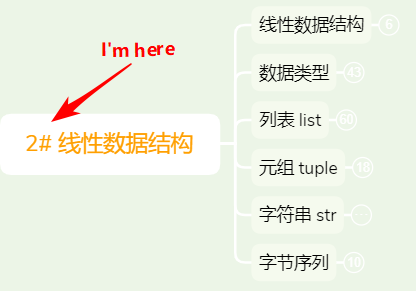# 1 线性数据结构

• 线性表：是一种抽象的数学概念，是一组元素的序列的抽象，由有穷个元素组成(0个或任意个)。

线性表又可分为 顺序表和链接表。

• 顺序表：一组元素在内存中有序的存储。列表list就是典型的顺序表。

• 链接表：一组元素在内存中分散存储链接起来，彼此知道连接的是谁。

对于这两种表，数组中的元素进行查找、增加、删除、修改，看看有什么影响：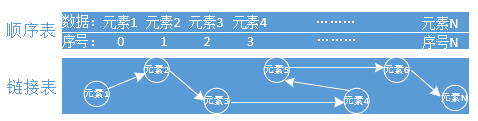• 查找元素

对于顺序表，是有序的在内存中存储数据，可快速通过索引编号获取元素，效率高。。

对于链接表是分散存储的，只能通过一个个去迭代获取元素，效率差。

• 增加元素

对于顺序表，如果是在末尾增加元素，对于整个数据表来说没什么影响，但是在开头或是中间插入元素，后面的所有元素都要重新排序，影响很大(想想数百万或更大数据量)。

对于链接表，不管在哪里加入元素，不会影响其他元素，影响小。

• 删除元素
对于顺序表，删除元素和增加元素有着一样的问题。
对于链接表，不管在哪里删除元素，不会影响其他元素，影响小。

• 修改元素
对于顺序表，可快速通过索引获取元素然后进行修改，效率高。

对于链接表，只能通过迭代获取元素然后进行修改，效率低。

# 2.内建常用的数据类型

## 2.1 数值型

• int 整数类型

说明：整数包括负整数、0、正整数（… -2,-1,0,1,2, …）。

``x1 = 1  x2 = 0  x3 = -1  print(type(x1), x1)  print(type(x2), x2)  print(type(x3), x3)    # 输出结果如下：  <class 'int'> 1  <class 'int'> 0  <class 'int'> -1  ``

int( )方法：可以将数字或字符串转为整数，缺省base=10,表示10进制，无参数传入则返回0。

``x1 = int()  x2 = int('1')  x3 = int('0b10',base=2)  #base=2，表二进制，与传入参数类型一致。  x4 = int(3.14)  print(type(x1), x1)  print(type(x2), x2)  print(type(x3), x3)  print(type(x4), x4)    # 输出结果如下：  <class 'int'> 0  <class 'int'> 1  <class 'int'> 2  <class 'int'> 3  ``
• float 浮点类型
说明：由整数和小数部分组成，传入的参数可以为`int``str``bytes``bytearray`

``x1 = float(1)  x2 = float('2')  x3 = float(b'3')  print(type(x1), x1)  print(type(x2), x2)  print(type(x3), x3)    # 输出结果如下：  <class 'float'> 1.0  <class 'float'> 2.0  <class 'float'> 3.0  ``
• complex (复数类型)

说明：由实数和虚数部分组成，都是浮点数。

传入参数可以为`int``str`，如果传入两参，前面一个为实数部分，后一个参数为虚数部分。

``x1 = complex(1)  x2 = complex(2,2)  x3 = complex('3')  print(type(x1), x1)  print(type(x2), x2)  print(type(x3), x3)    # 输出结果如下：  <class 'complex'> (1+0j)  <class 'complex'> (2+2j)  <class 'complex'> (3+0j)  ``
• bool (布尔类型)

说明：为int的子类，返回的是True和False，对应的是1和0。

``x1 = bool(0)  x2 = bool(1)  x3 = bool()  x4 = 2 > 1  print(type(x1), x1)  print(type(x2), x2)  print(type(x3), x3)  print(type(x4), x4)    # 输出结果如下：  <class 'bool'> False  <class 'bool'> True  <class 'bool'> False  <class 'bool'> True  ``

## 2.2 序列(sequence)

### 2.2.1 list 列表

• 初始化

``lst = []  # 空列表方式1  #或者  lst = list()  # 空列表方式2  print(type(lst),lst)    # 输入结果如下：  <class 'list'> []  ``
• 索引

说明： 使用正索引(从左至右)、负索引(从右至左)访问元素，时间复杂度为`O(1)`，效率极高的使用方式。

按照给定区间获取到数据，叫做切片。

正索引：

从左至右，从0开始索引，区间为[0，长度-1]，左包右不包。

``lst = ['a','b','c','d']  print(lst)  # 获取第一个元素  print(lst[1:2])  # 获取第二个元素,左包右不包，切片  print(lst[2:])  # 获取第三个元素到最后一个元素，切片  print(lst[:])  # 获取所有元素，切片    # 输出结果如下：  a  ['c']  ['c', 'd']  ['a', 'b', 'c', 'd']  ``

负索引：

从右至左，从-1开始索引，区间为[-长度，-1]

``lst = ['a','b','c','d']  print(lst[-1])  print(lst[-2:])    # 输出结果如下：  d  ['c', 'd']  ``
• 查询

index( )方法：`L.index(value, [start, [stop]]) -> integer`

返回的是索引id，要迭代列表，时间复杂度为O(n)。

``lst = ['a','b','c','d']  print(lst.index('a',0,4))  # 获取区间[0,4]的元素'a'的索引id    # 输出结果如下：  0  ``

备注：如果查询不到元素，则抛出`ValueError`

count( ) 方法：L.count(value) -> integer

返回的是元素出现的次数，要迭代列表，时间复杂度为O(n)。

``lst = ['a','b','a','b']  print(lst.count('a'))    # 输出结果如下：  2  ``

len( ) 方法：返回的是列表元素的个数，时间复杂度为O(1)。

``lst = ['a','b','c','d']  print(len(lst))    # 输出结果如下：  4  ``

备注：所谓的O(n) 是指随着数据的规模越来越大，效率下降，而O(1)则相反，不会随着数据规模大而影响效率。

• 修改

列表是有序可变，所以能够对列表中的元素进行修改。

``lst = ['a','b','c','d']  lst = 'A'  print(lst)    # 输出结果如下：  ['A', 'b', 'c', 'd']  ``
• 增加

append( ) 方法：`L.append(object) -> None`

尾部追加元素，就地修改，返回None。

``lst = ['a','b','c','d']  lst.append('e')  print(lst)    # 输出结果如下：  ['a', 'b', 'c', 'd', 'e']  ``

insert( )方法：`L.insert(index, object) -> None` ,

在指定索引位置插入元素对象，返回None。

``lst = ['a','b','c','d']  lst.insert(0,'A')  # 在索引0位置插入'A',原有的元素全部往后移，增加了复杂度  print(lst)    # 输出结果如下：  ['A', 'a', 'b', 'c', 'd']  ``

extend( )方法： `L.extend(iterable) -> None`

可以增加多个元素，将可迭代对象的元素追加进去，返回None。

``lst = ['a','b','c','d']  lst.extend([1,2,3])  print(lst)    # 输出结果如下：  ['a', 'b', 'c', 'd', 1, 2, 3]  ``

还可以将列表通过 `+``*` ，拼接成新的列表。

``lst1 = ['a','b','c','d']  lst2 = ['e','f','g']  print(lst1 + lst2)  print(lst1 * 2)  # 将列表里面的元素各复制2份    # 输出结果如下：  ['a', 'b', 'c', 'd', 'e', 'f', 'g']  ['a', 'b', 'c', 'd', 'a', 'b', 'c', 'd']  ``

这里还有一个特别要注意情况如下：

``lst1 = [] * 3  # 结果：[, , ]  print(lst1)  lst1 = 10  # 结果：[, , ]，是这样嘛？？  print(lst1)    # 输出结果如下：  [, , ]  [, , ]  # 为什么结果会是这个？请往下看列表复制章节，找答案！  ``
• 删除

remove()方法：`L.remove(value) -> None`

从左至右遍历查找，找到就删除该元素，返回None，找不到则抛出`ValueError`

``lst = ['a','b','c','d']  lst.remove('d')  print(lst)    # 输出结果如下：  ['a', 'b', 'c']  # 元素'd'已经被删除  ``

pop() 方法：`L.pop([index]) -> item`

缺省删除尾部元素，可指定索引删除元素，索引越界抛出`IndexError`

``lst = ['a','b','c','d']  lst.pop()  print(lst)    # 输出结果如下：  ['a', 'b', 'c']  ``

clear() 方法：`L.clear() -> None`

清空列表所有元素，慎用。

``lst = ['a','b','c','d']  lst.clear()  print(lst)    # 输出结果如下：  []  # 空列表了  ``
• 反转

reverse( ) 方法：`L.reverse()`

将列表中的元素反转，返回None。

``lst = ['a','b','c','d']  lst.reverse()  print(lst)    # 输出结果如下：  ['d', 'c', 'b', 'a']  ``
• 排序

sort() 方法：`L.sort(key=None, reverse=False) -> None`

对列表元素进行排序，缺省为升序，reverse=True为降序。

``lst = ['a','b','c','d']  lst.sort(reverse=True)  print(lst)    # 输出结果如下：  ['d', 'c', 'b', 'a']  ``
• in成员操作

判断成员是否在列表里面，有则返回True、无则返回False。

``lst = ['a','b','c','d']  print('a' in lst)  print('e' in lst)    # 输出结果如下：  True  False  ``
• 列表复制

说明： 列表复制指的是列表元素的复制，可分为浅copy和深copy两种。列表元素对象如列表、元组、字典、类、实例这些归为引用类型(指向内存地址)，而数字、字符串先归为简单类型，好让大家理解。

示例一：这是属于拷贝嘛？

``lst1 = [1,[2,3],4]  lst2 = lst1  print(id(lst1),id(lst2),lst1 == lst2, lst2)  # id() 查看内存地址    # 输出结果如下：  1593751168840 1593751168840 True [1, [2, 3], 4]  ``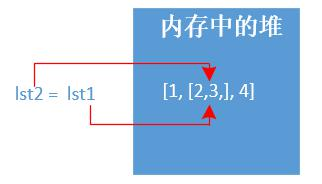显然不是属于任何copy，说白了都是指向同一个内存地址。

示例二：浅拷贝copy

说明： 浅拷贝对于`引用类型`对象是不会copy的，地址指向仍是一样。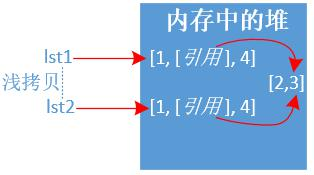``lst1 = [1,[2,3],4]  lst2 = lst1.copy()  print(id(lst1),id(lst2),lst1 == lst2, lst2)  print('=' * 30)  lst1 = 200  # 修改列表的引用类型，所有列表都会改变  print(lst1, lst2)    # 输出结果如下：  1922175854408 1922175854344 True [1, [2, 3], 4]  ==============================  [1, [200, 3], 4] [1, [200, 3], 4]  ``

示例三：深拷贝deepcopy

说明： 深拷贝对于`引用类型`对象也会copy成另外一份，地址指向不一样。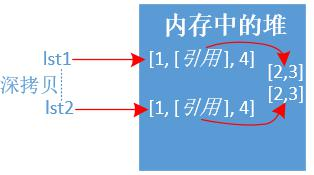``import copy    lst1 = [1,[2,3],4]  lst2 = copy.deepcopy(lst1)  print(id(lst1),id(lst2),lst1 == lst2, lst2)  print('=' * 30)  lst1 = 200  # 修改列表的引用类型，不会影响其他列表  print(lst1, lst2)    # 输出结果如下：  2378580158344 2378580158280 True [1, [2, 3], 4]  ==============================  [1, [200, 3], 4] [1, [2, 3], 4]  ``

### 2.2.2 tuple 元组

• 初始化

``t1 = ()  # 空元素方式1，一旦创建将不可改变  t2 = tuple()  # 空元素方式2，一旦创建将不可改变  t3 = ('a',)  # 元组只有一个元素，一定要加逗号','  t4 = (['a','b','c'])  # 空列表方式2  ``

备注： 元组如果只有一个元素对象，一定要在后面加逗号`,` 否则变为其他数据类型。

• 索引
同列表一样，不再过多举例。

``t = ('a','b','c','d')  print(t)  print(t[-1])  # 输出结果如下：  a  d  ``
• 查询

同列表一样，不再过多举例。

``t = ('a','b','c','d')  print(t.index('a'))  print(t.count('a'))  print(len(t))    # 输出结果如下：  0  1  4  ``
• 增删改

元组是`不可变`类型，不能增删改元素对象。

但是要注意如下场景：

元组中的元素对象(内存地址)不可变，引用类型可变。—-这里又出现引用类型的情况了。

``# 元组的元组不可修改(即内存地址)  t = (,)  t= 100  print(t)  # 结果报错了  TypeError: 'tuple' object does not support item assignment    ############################################    # 元组里面的引用类型对象可以修改（如嵌套了列表）  t = (,2,3)  t = 100  # 对元组引用类型对象的元素作修改  print(t)    # 输出结果如下：  (, 2, 3)  ``

### 2.2.3 string 字符串

• 初始化
多种花样，使用单引号、双引号、三引号等。

``name = 'tom'  age = 18  str1 = 'abc'  # 单引号字符串  str2 = "abc"  # 双引号字符串  str3 = """I'm python"""  # 三引号字符串  str4 = r"c:windowsnote"  # r前缀，没有转义(转义字符不生效)  str5 = f'{name} is {age} age.'  # f前缀，字符串格式化，v3.6支持  print(type(str1), str1)  print(type(str2), str2)  print(type(str3), str3)  print(type(str4), str4)  print(type(str5), str5)    # 输出结果如下：  <class 'str'> abc  <class 'str'> abc  <class 'str'> I'm python  <class 'str'> c:windowsnote  <class 'str'> tom is 18 age.  ``
• 索引

同列表一样，不再过多举例。

``str = "abcdefg"  print(str)  print(str[-1])    # 输出结果如下：  a  g  ``
• 连接

通过加号 `+` 将多个字符串连接起来，返回一个新的字符串。

``str1 = "abcd"  str2 = "efg"  print(str1 + str2)    # 输出结果如下：  abcdefg  ``

join( ) 方法：`S.join(iterable) -> str`

s表示分隔符字符串，iterable为可迭代对象字符串，结果返回字符串。

``str = "abcdefg"  print('->'.join(str))    # 输出结果如下：  a->b->c->d->e->f->g  ``
• 字符查找

find( ) 方法：`S.find(sub[, start[, end]]) -> int`

从左至右查找子串sub，也可指定区间，找到返回正索引，找不到则返回 `-1`

``str = "abcdefg"  print(str.find('a',0,7))  print(str.find('A'))    # 输出结果如下：  0  -1  ``

rfind( ) 方法：`S.rfind(sub[, start[, end]]) -> int`

从右至左查找子串sub，也可指定区间，找到返回正索引，找不到则返回 `-1`

``str = "abcdefg"  print(str.rfind('a'))  print(str.rfind('A'))    # 输出结果如下：  0  -1  ``

还有`index()``find()` 类似，不过找不到会抛异常，不建议使用。

`s.count()` 还可以统计字符出现的次数。

`len(s)` 还可以统计字符串的长度。

• 分割

split( ) 方法：`S.split(sep=None, maxsplit=-1) -> list of strings`

sep表示分隔符，缺省为空白字符串，maxsplit=-1表示遍历整个字符串，最后返回列表。

``str = "a,b,c,d,e,f,g"  print(str.split(sep=','))    # 输出结果如下：  ['a', 'b', 'c', 'd', 'e', 'f', 'g']  ``

rsplit( ) 方法与上面不同就是，从右至左遍历。

splitlines() 方法： `S.splitlines([keepends]) -> list of strings`

按行来切割字符串，keepends表示是否保留行分隔符，最后返回列表。

``str = "anbncrnd"  print(str.splitlines())  print(str.splitlines(keepends=True))    # 输出结果如下：  ['a', 'b', 'c', 'd']  ['an', 'bn', 'crn', 'd']  ``

partition() 方法`S.partition(sep) -> (head, sep, tail)`

从左至右查询分隔符，遇到就分割成头、分隔符、尾的三元组，返回的是一个元组tuple。

``str = "a*b*c*d"  print(str.partition('*'))  # 输出结果如下：  ('a', '*', 'b*c*d')  ``

rpartition() 方法`S.rpartition(sep) -> (head, sep, tail)`

与上方法不同，就是从右至左，不过这个比较常用，可以获取后缀部分信息。

``str1 = "http://www.python.org:8843"  str2 = str1.rpartition(':')  port = str2[-1]  print(port)  ``
• 替换

replace() 方法：`S.replace(old, new[, count]) -> str`

遍历整个字符串，找到全部替换，count表示替换次数，缺省替换全部，最后返回一个`新的字符串`

``str = "www.python.org"  print(str.replace('w','m'))  # 返回的是一个新的字符串  print(str)  # 字符串不可变，保持原样    # 输出结果如下：  mmm.python.org  www.python.org  ``
• 移除

strip() 方法：`S.strip([chars]) -> str`

在字符串两端移除指定的`字符集chars` , 缺省移除空白字符。

``str = " * www.python.org  *"  print(str.strip("* "))  # 去掉字符串首尾带有星号'*' 和 空白' '    # 输出结果如下：  www.python.org  ``

还有`lstrip()``rstrip` 分别是移除字符串左边和右边字符集。

• 首尾判断

startswith() 方法：`S.startswith(prefix[, start[, end]]) -> bool`

缺省判断字符串开头是否有指定的字符prefix,也可指定区间。

``str = "www.python.org"  print(str.startswith('www',0,14))  print(str.startswith('p',0,14))  # 输出结果如下：  True  False  ``

endswith() 方法：`S.endswith(suffix[, start[, end]]) -> bool`

缺省判断字符串结尾是否有指定的字符suffix,也可指定区间。

``str = "www.python.org"  print(str.startswith('www',0,14))  print(str.startswith('p',0,14))  # 输出结果如下：  True  False  ``
``str = "www.python.org"  print(str.endswith('g',11,14))  # 输出结果如下：  True  ``
• 格式化

c风格格式化：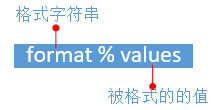格式字符串：使用%s(对应值为字符串)，%d(对应值为数字)等等，还可以在中间插入修饰符%03d。

被格式的值：只能是一个对象，可以是元组或是字典。

``name = "Tom"  age = 18  print("%s is %d age." % (name,age))  # 输出结果如下：  Tom is 18 age.  ``

format格式化：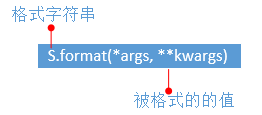格式字符串：使用花括号{ }, 花括号里面可以使用修饰符。

被格式的值：*args为可变位置参数，**kwargs为可变关键字参数。

``# 位置传参  print("IP={} PORT={}".format('8.8.8.8',53))  # 位置传参  print("{Server}: IP={1} PORT={0}".format(53, '8.8.8.8', Server='DNS Server'))  # 位置和关键字传参传参    # 输出结果如下：  IP=8.8.8.8 PORT=53  DNS Server: IP=8.8.8.8 PORT=53  ``
``# 浮点数  print("{}".format(0.123456789))  print("{:f}".format(0.123456789))    #  小数点默认为6位  print("{:.2f}".format(0.123456789))  # 取小数点后两位  print("{:15}".format(0.123456789))   # 宽度为15，右对齐    # 输出结果如下：  0.123456789  0.123457     # 为什么是这个值？大于5要进位  0.12      0.123456789  # 左边有4个空格  ``
• 其他常用函数

``str = "DianDiJiShu"  print(str.upper())  # 字母全部转化为大写  print(str.lower())  # 字母全部转化为小写    # 输出结果如下：  DIANDIJISHU  diandijishu  ``

### 2.2.4 bytes 字节

`bytes ``bytearray`从python3引入的两种数据类型。

• 把人类的语言编码成机器能够识别的语言，通常叫做编码（字符串转换为ASCII码）。
• 把机器的语言解码成人类能够识别的语言，通常叫做解码（ASCII码转换为字符串）。

ASCII码表常用的必须牢记(整理部分)：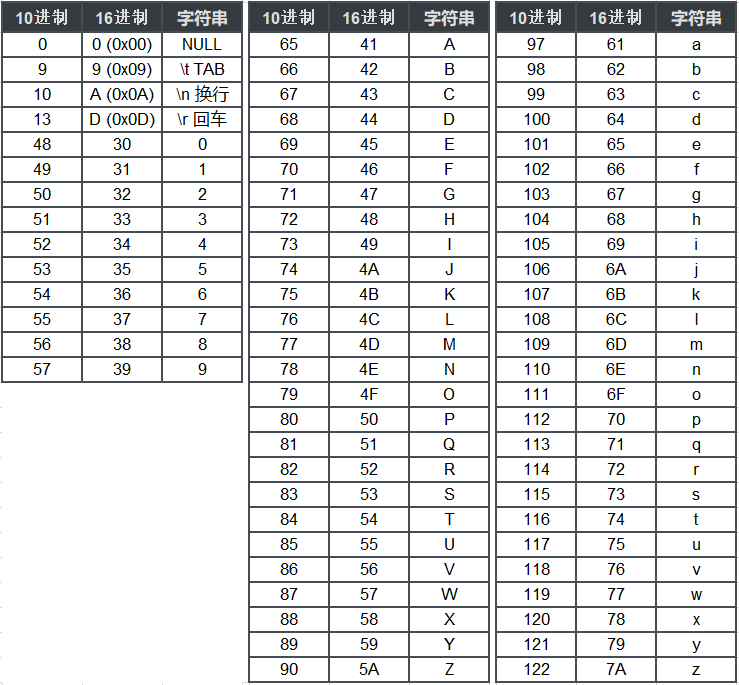``链接：https://pan.baidu.com/s/1fWVl57Kqmv-tkjrDKwPvSw 提取码：tuyz  ``

``# 二进制  0000 0010  # 一个字节bytes    # 16进制，机器基本都是显示16进制  0x2  ``

bytes 是不可变类型

``bytes()     # 空bytes，一旦创建不可改变  bytes(int)  # 指定字节的大小，用0填充  bytes(iterable_of_ints)  # [0.255]整数的可迭代对象  bytes(string, encoding[, errors])  # 等价于string.encoding(),字符串编码成字节  bytes(bytes_or_buffer)  # 复制一份新的字节对象  ``
• 初始化

``b1 = bytes()  b2 = bytes(range(97,100))  b3 = bytes(b2)  b4 = bytes('123',encoding='utf-8')  b5 = b'ABC'  b6 = b'xe4xbdxa0xe5xa5xbd'.decode('utf-8')  print(b1, b2, b3, b4, b5, b6, sep='n')    # 输出结果如下：  b''  b'abc'  b'abc'  b'123'  b'ABC'  你好  ``

### 2.2.5 bytearray 字节数组

bytearray 是可变数组，可以进行增删改操作，类似列表。

``bytearray()  # 空bytearray,可改变  bytearray(iterable_of_ints)  # [0.255]整数的可迭代对象  bytearray(string, encoding[, errors])  # 等价于string.encoding(),字符串编码成字节  bytearray(bytes_or_buffer)   # 复制一份新的字节数组对象  bytearray(int)  # 指定字节的大小，用0填充  ``
• 增删改

``# 初始化  b = bytearray()  print(b)  # 输出结果如下：  bytearray(b'')  #--------------------------  # 增加元素对象  b.append(97)  print(b)  b.extend([98,99])  print(b)  # 输出结果如下：  bytearray(b'a')  bytearray(b'abc')  #--------------------------  # 插入元素对象  b.insert(0,65)  print(b)  # 输出结果如下：  bytearray(b'Aabc')  #--------------------------  # 删除元素对象  b.pop()  print(b)  # 输出结果如下：  bytearray(b'Aab')  ``